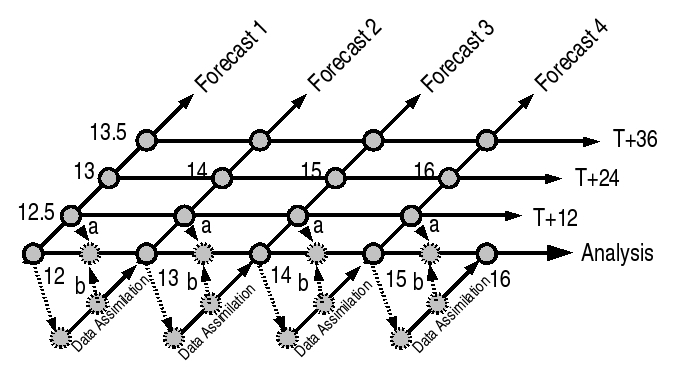My first post at describing the time issues for meteorological metadata led to some confusion, so I’m trying again. I think it helps to consider a diagram:The diagram shows a number of different datasets that can be constructed from daily forecast runs (shown for an arbitrary month from the 12th til the 15th as forecasts 1 through 4. If we consider forecast 2, we are running it to give me a forecast from the analysis time (day 13.0) forward past day 14.5 … but you can see that the simulation began (in this case 1.0) days earlier, at a simulation time of 12.0 (T0). In this example

• we’ve allowed the initial condition to be day 12.0 from forecast 1.
• we’ve imagined the analysis was produced at the end of a data assimilation period (let’s call this time Ta).
• the last time for which data was used (the datum time, td) corresponds to the analysis time.

(Here I’m using some nomenclature defined earlier as well as using some new terms).

Anyway, the point here was to introduce a couple of new concepts. These forecast datasets can be stored and queried relatively simply … we would have a sequence of datasets, one for each forecast, and the queries would simply then be on finding the forecasts (using discovery metadata, e.g. ISO19139) and then on extracting and using the data itself (using archive aka content aka usage metadata, e.g. an application schema of GML such as CSML).

What’s more interesting is how we provide, document and query the sythesized datasets (e.g. the analysis and T+24 datasets). Firstly, if we look at the analysis dataset, we could extract the Ta data points and have a new dataset, but often we need the interim times as well, and you can see that we have two choices of how to construct them - we can use the earlier time from the later forecast (b), or the later time from the earlier forecast (a). Normally we choose the latter, because the diabatic and non-observed variables are usually more consistent outside the assimilation period when they have had longer to spin up. Anyway, either way we have to document what is done. This is a job for a new package we plan to get into the WMO core profile of ISO19139 as an extension - NumSim.

From a storage point of view, as I implied above, we can extract and store the new datasets, or we can try and do this as a virtual dataset, described in CSML, and extractable by CSML-tools. We don’t yet know how to do this, but there is obvious utility in saving storage in doing so.